# ML Aggarwal Class 10 Solutions for ICSE Maths Chapter 17 Mensuration MCQS

## ML Aggarwal Class 10 Solutions for ICSE Maths Chapter 17 Mensuration MCQS

These Solutions are part of ML Aggarwal Class 10 Solutions for ICSE Maths. Here we have given ML Aggarwal Class 10 Solutions for ICSE Maths Chapter 17 Mensuration MCQS

More Exercise

Choose the correct answer from the given four options (1 to 32) :

Question 1.
In a cylinder, if radius is halved and height is doubled then the volume will be
(a) same
(b) doubled
(c) halved
(d) four times
Solution:
Let radius of cylinder = r
and height = h
then volume = πr²h
If the radius is halved and the height is doubled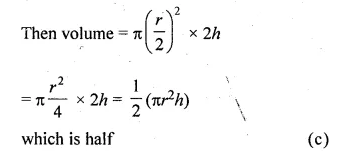Question 2.
In a cylinder, if the radius is doubled &nd height is halved then its curved surface area will be
(a) halved
(b) doubled
(c) same
(d) four times
Solution:
Let radius of a cylinder = r
and height = h
Then curved surface area = 2πrh
Now if radius is doubled and height is halved,
then curved surface area = 2π$$\\ \frac { r }{ 2 }$$ × 2h = 2πrh
which is same (c)

Question 3.
If a well of diameter 8 m has been dug to the depth of 14 m, then the volume of the earth dug out is
(a) 352 m3
(b) 704 m3
(c) 1408 m3
(d) 2816 m3
Solution:
Diameter of a well = 8 m
Radius (r) = $$\\ \frac { 8 }{ 2 }$$ = 4m
Depth (h) = 14 m
Volume of the earth dug put = πr2h
= $$\\ \frac { 22 }{ 7 }$$ × 4 × 4 × 14 m3
= 704 m3 (b)

Question 4.
If two cylinders of the same lateral surface have their radii in the ratio 4 : 9, then the ratio of their heights is
(a) 2 : 3
(b) 3 : 2
(c) 4 : 9
(d) 9 : 4
Solution:
Ratio in two cylinder having same lateral surface area their radii is 4 : 9
Let r1 be the radius of the first and r2 be the second cylinder
and h1, h2 and their heights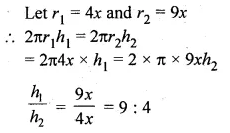Ratio in their heights = 9 : 4 (d)

Question 5.
The radii of two cylinders are in the ratio 2 : 3 and their heights are in the ratio 5 : 3. The ratio of their volumes is
(a) 10 : 17
(b) 20 : 27
(c) 17 : 27
(d) 20 : 37
Solution:
Radii of two cylinder are in the ratio = 2 : 3
Ratio in their height = 5 : 3
Let height of the first cylinder = 5y
and of second = 3y
Now, volume of the first cylinder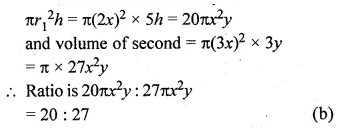Question 6.
The total surface area of a cone whose radius is $$\\ \frac { r }{ 2 }$$ and slant height 2l is
(a) 2πr (l + r)
(b) $$\pi r\left( l+\frac { r }{ 4 } \right)$$
(c) πr(l + r)
(d) 2πrl
Solution:
Radius of a cone = $$\\ \frac { r }{ 2 }$$
and slant height = 2l
total surface area of a cone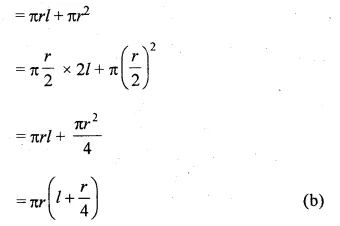Question 7.
If the diameter of the base of cone is 10 cm and its height is 12 cm, then its curved surface area is
(a) 60π cm2
(b) 65π cm2
(c) 90π cm2
(d) 120π cm2
Solution:
Diameter of the base of a cone = 10 cm
Radius (r) = $$\\ \frac { 10 }{ 2 }$$ = 5 cm
and height (h) = 12 cm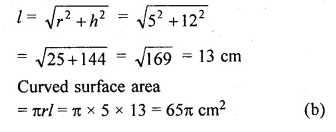Question 8.
If the diameter of the base of a cone is 12 cm and height is 20 cm, then its volume is ,
(a) 240π cm3
(b) 480π cm3
(c) 720π cm3
(d) 960π cm3
Solution:
Diameter of the base of a cone = 12 cm
Radius (r) = $$\\ \frac { 12 }{ 2 }$$ = 6 cm
and height (h) = 20 cm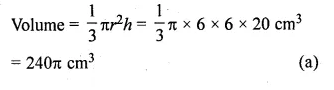Question 9.
If the radius of a sphere is 2r, then its volume will be
(a) $$\frac { 4 }{ 3 } \pi { r }^{ 3 }$$
(b) $$4\pi { r }^{ 3 }$$
(c) $$\frac { 8\pi { r }^{ 3 } }{ 3 }$$
(d) $$\frac { 32\pi { r }^{ 3 } }{ 3 }$$
Solution:
Radius of a sphere = 2r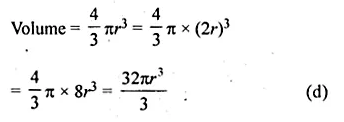Question 10.
If the diameter of a sphere is 16 cm, then its surface area is
(a) 64π cm2
(b) 256π cm2
(c) 192π cm2
(d) 256 cm2
Solution:
Diameter of a sphere = 16 cm
Radius (r) = $$\\ \frac { 16 }{ 2 }$$ = 8 cm
Surface area = 4π2 = 4π × 8 × 8 cm2 = 256π cm2 (b)

Question 11.
If the radius of a hemisphere is 5 cm, then its volume is
(a) $$\frac { 250 }{ 3 } \pi { \quad cm }^{ 3 }$$
(b) $$\frac { 500 }{ 3 } \pi { \quad cm }^{ 3 }$$
(c) $$75\pi { \quad cm }^{ 3 }$$
(d) $$\frac { 125 }{ 3 } \pi { \quad cm }^{ 3 }$$
Solution:
Radius of a hemisphere (r) = 5 cm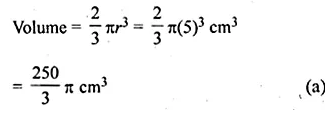Question 12.
If the ratio of the diameters of the two spheres is 3 : 5, then the ratio of their surface areas is
(a) 3 : 5
(b) 5 : 3
(c) 27 : 125
(d) 9 : 25
Solution:
Ratio in the diameters of two spheres = 3 : 5
Let radius of the first sphere = 3x cm
and radius of the second sphere = 5x cm
Ratio in their surface area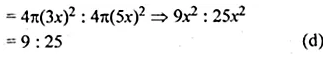Question 13.
The radius of a hemispherical balloon increases from 6 cm to 12 cm as air is being pumped into it. The ratio of the surface areas of the balloon in the two cases is
(a) 1 : 4
(b) 1 : 3
(c) 2 : 3
(d) 2 : 1
Solution:
Radius of balloon (hemispherical) in the original position = 6 cm
and in increased position = 12 cm
Ratio in their surface areas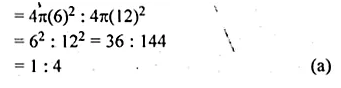Question 14.
The shape of a Gilli, in the game of Gilli- danda, is a combination of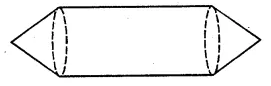(a) two cylinders
(b) a cone and a cylinders
(c) two cones and a cylinder
(d) two cylinders and a cone
Solution:
The shape of a Gilli is the combination of
two cones and a cylinder (as shown in the figure). (c)

Question 15.
If two solid hemisphere of same base radius r are joined together along with their bases, then the curved surface of this new solid is
(a) 4πr2
(b) 6πr2
(c) 3πr2
(d) 8πr2
Solution:
Radius of two solid hemispheres = r
These are joined together along with the bases
Curved surface area = 2π2 × 2 = 4πr2 (a)

Question 16.
During conversion of a solid from one shape to another, the volume of the new shape will
(a) increase
(b) decrease
(c) remain unaltered
(d) be doubled
Solution:
During the conversion of a solid into another,
the volume of the new shaper will be the same.
i.e. remain unaltered (c)

Question 17.
If a solid of one shape is converted to another, then the surface area of the new solid
(a) remains same
(b) increases
(c) decreases
(d) can’t say
Solution:
If a solid of one shape has conversed into another then
the surface area of the new solid will same or not same
i.e. can’t say. (d)

Question 18.
If a marble of radius 2.1 cm is put into a cylindrical cup full of water of radius 5 cm and height 6 cm, then the volume of water that flows out of the cylindrical cup is
(a) 38.8 cm3
(b) 55.4 cm3
(c) 19.4 cm3
(d) 471.4 cm3
Solution:
Radius of a marble = 2.1 cm
Volume of marble = $$\frac { 4 }{ 3 } \pi { { r }^{ 3 }\quad cm }^{ 3 }$$
= $$\\ \frac { 4 }{ 3 }$$ x $$\\ \frac { 22 }{ 7 }$$ × 2.1 × 2.1 × 2.1 cm3
= 38.88 cm3
= 38.8 cm3 (a)

Question 19.
The volume of the largest right circular cone that can be carved out from a cube of edge 4.2 cm is
(a) 9.7 cm3
(b) 77.6 cm3
(c) 58.2 cm3
(d) 19.4 cm3
Solution:
Edge of cube = 4.2 cm
Radius of largest cone cut out = $$\\ \frac { 42.2 }{ 2 }$$ = 2.1 cm
and height = 4.2 cm
Volume = $$\frac { 1 }{ 3 } \pi { { r }^{ 2 }h }$$
= $$\\ \frac { 1 }{ 3 }$$ x $$\\ \frac { 22 }{ 7 }$$ × 2.1 × 2.1 × 4.2 cm3
= 19.404
= 19.4 cm3 (d)

Question 20.
The volume of the greatest sphere cut off from a circular cylindrical wood of base radius 1 cm and height 6 cm is
(a) 288 π cm3
(b) $$\frac { 4 }{ 3 } \pi$$ cm3
(c) 6 π cm3
(d) 4 π cm3
Solution:
Radius of cylinder (r) = 1 cm
Height (h) = 6 cm
The largest sphere that can be cut off from the cylinder of radius 1 cm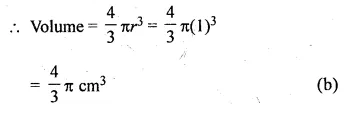Question 21.
The volumes of two spheres are in the ratio 64 : 27. The ratio of their surface areas is
(a) 3 : 4
(b) 4 : 3
(c) 9 : 16
(d) 16 : 9
Solution:
Ratio in volumes of two spheres = 64 : 27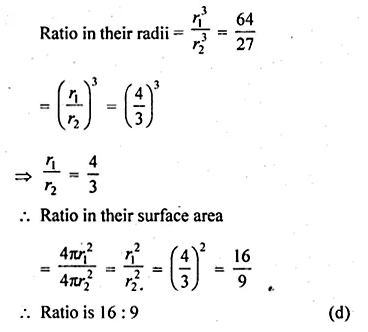Question 22.
If a cone, a hemisphere and a cylinder have equal bases and have same height, then the ratio of their volumes is
(a) 1 : 3 : 2
(b) 2 : 3 : 1
(c) 2 : 1 : 3
(d) 1 : 2 : 3
Solution:
If a cone, a hemisphere and a cylinder have equal bases = r (say)
and height = h in each case and r = h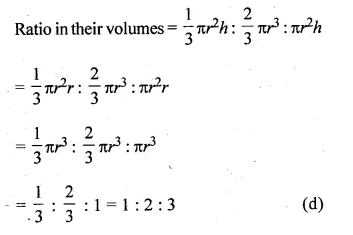Question 23.
If a sphere and a cube have equal surface areas, then the ratio of the diameter of the sphere to the edge of the cube is
(a) 1 : 2
(b) 2 : 1
(c) √π : √6
(d) √6 : √π
Solution:
A sphere and a cube have equal surface area
Let a be the edge of a cube and r be the radius of the sphere, then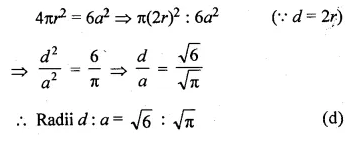Question 24.
A solid piece of iron in the form of a cuboid of dimensions 49 cm x 33 cm x 24 cm is moulded to form a sphere. The radius of the sphere is
(a) 21 cm
(b) 23 cm
(c) 25 cm
(d) 19 cm
Solution:
Dimension of a cuboid = 49 cm × 33 cm × 24 cm
Volume of a cuboid = 49 × 33 × 24 cm3
⇒ Volume of sphere = Volume of a cuboid
Volume of a sphere = 49 × 33 × 24 cm3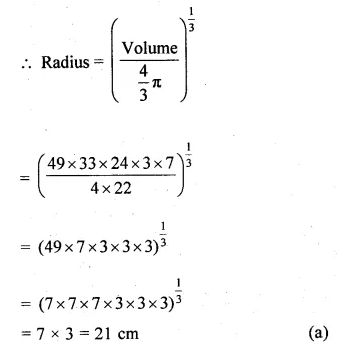Question 25.
If a solid right circular cone of height 24 cm and base radius 6 cm is melted and recast in the shape of a sphere, then the radius of the sphere is
(a) 4 cm
(b) 6 cm
(c) 8 cm
(d) 12 cm
Solution:
Height of a circular cone (h) = 24 cm
and radius (r) = 6 cm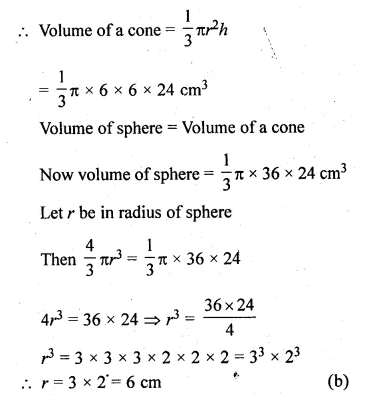Question 26.
If a solid circular cylinder of iron whose diameter is 15 cm and height 10 cm is melted and recasted into a sphere, then the radius of the sphere is
(a) 15 cm
(b) 10 cm
(c) 7.5 cm
(d) 5 cm
Solution:
Diameter of a cylinder = 15 cm
Radius = $$\\ \frac { 15 }{ 2 }$$ cm
and height = 10 cm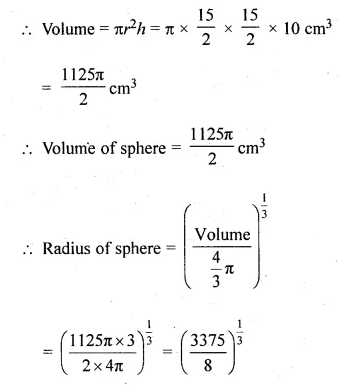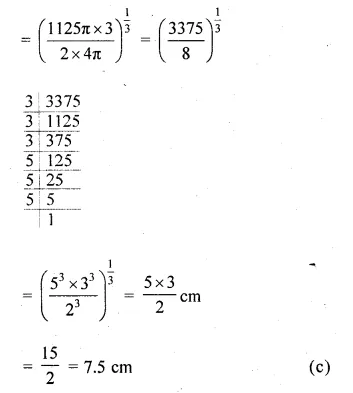Question 27.
The number of balls of radius 1 cm that can be made from a sphere of radius 10 cm is
(a) 100
(b) 1000
(c) 10000
(d) 100000
Solution:
Radius of sphere (R) = 10 cm
Volume of sphere = $$\frac { 4 }{ 3 } \pi { R }^{ 3 }=\frac { 4 }{ 3 } \pi { (10) }^{ 3 }{ cm }^{ 3 }$$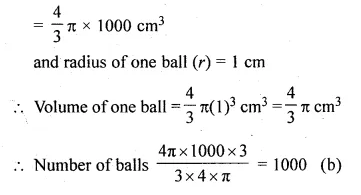Question 28.
A metallic spherical shell of internal and external diameters 4 cm and 8 cm, respectively is melted and recast into the form of a cone of base diameter 8 cm. The height of the cone is
(a) 12 cm
(b) 14 cm
(c) 15 cm
(d) 18 cm
Solution:
The internal diameter of the metallic shell = 4 cm
and external diameter = 8 cm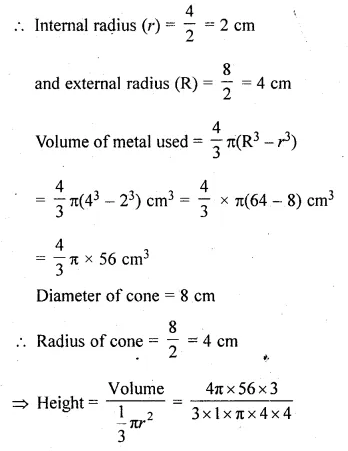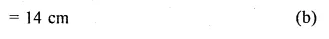Question 29.
A cubical icecream brick of edge 22 cm is to be distributed among some children by filling icecream cones of radius 2 cm and height 7 cm upto its brim. The number of children who will get the icecream cones is
(a) 163
(b) 263
(c) 363
(d) 463
Solution:
Edge of a cubical icecream brick = 22 cm
Volume = a3 = (22)3 = 10648 cm3
Radius (r) of ice cream cone (r) = 2 cm
and height (h) = 7 cm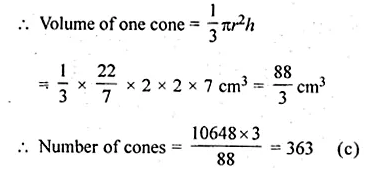Question 30.
Twelve solid spheres of the same size are made by melting a solid metallic cylinder of base diameter 2 cm and height 16 cm. The diameter of each sphere is
(a) 4 cm
(b) 3 cm
(b) 2 cm
(d) 6 cm
Solution:
Diameter of cylinder = 2 cm
Radius = $$\\ \frac { 2 }{ 2 }$$ = 1 cm
and height = 16 cm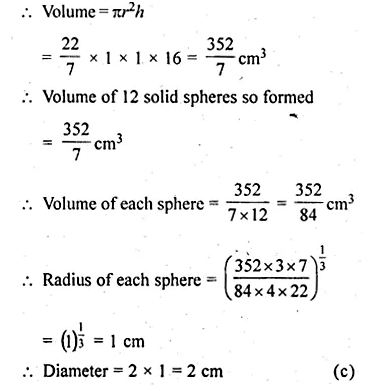Question 31.
A hollow cube of internal edge 22 cm is filled with spherical marbles of diameter 0.5 cm and it is assumed that $$\\ \frac { 1 }{ 8 }$$ space of the cube remains unfilled. Then the number of marbles that the cube can accommodate is
(a) 142296
(b) 142396
(c) 142496
(d) 142596
Solution:
Internal edge of a hollow cube = 22 cm
Volume = (side)3 = (22)3 = 22 × 22 × 22 cm3 = 10648 cm3
Diameter of spherical marble = 0.5 cm = $$\\ \frac { 1 }{ 2 }$$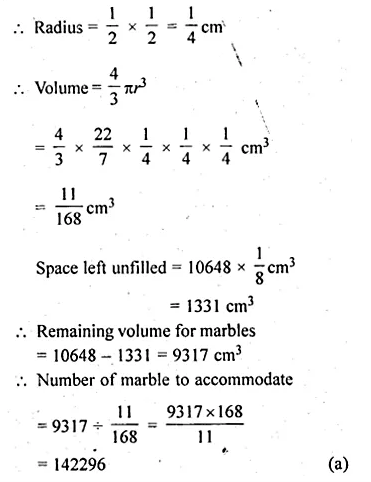Question 32.
In the given figure, the bottom of the glass has a hemispherical raised portion. If the glass is filled with orange juice, the quantity of juice which a person will get is
(a) 135 π cm3
(b) 117 π cm3
(c) 99 π cm3
(d) 36 π cm3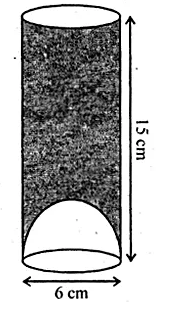Solution:
Radius of base of cylinder (r) = $$\\ \frac { 6 }{ 2 }$$ cm = 3 cm
and height (h)= 15 cm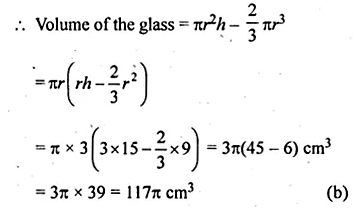We hope the ML Aggarwal Class 10 Solutions for ICSE Maths Chapter 17 Mensuration MCQS help you. If you have any query regarding ML Aggarwal Class 10 Solutions for ICSE Maths Chapter 17 Mensuration MCQS, drop a comment below and we will get back to you at the earliest.